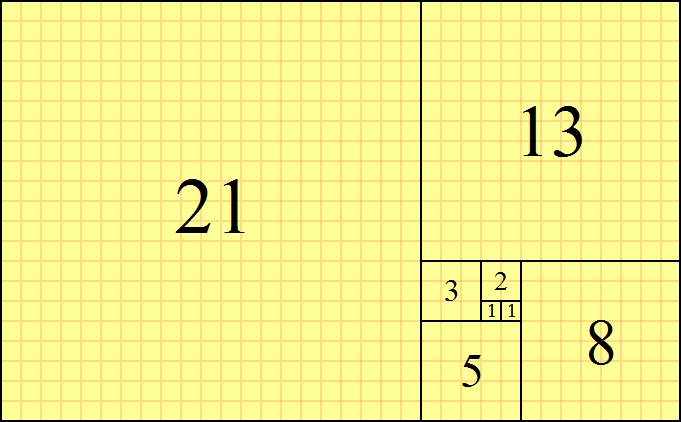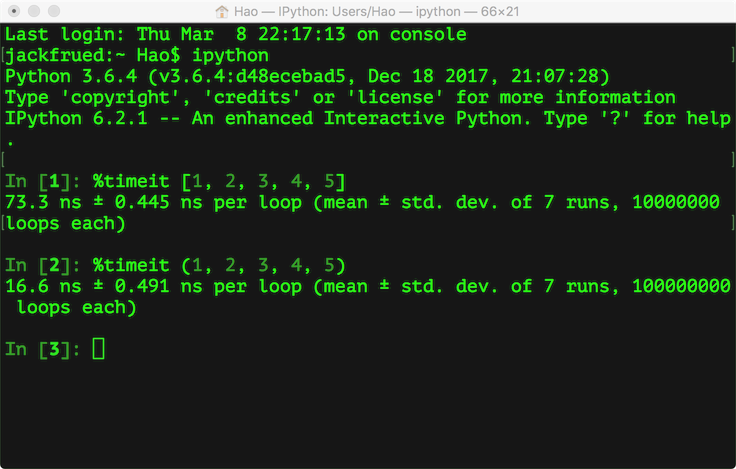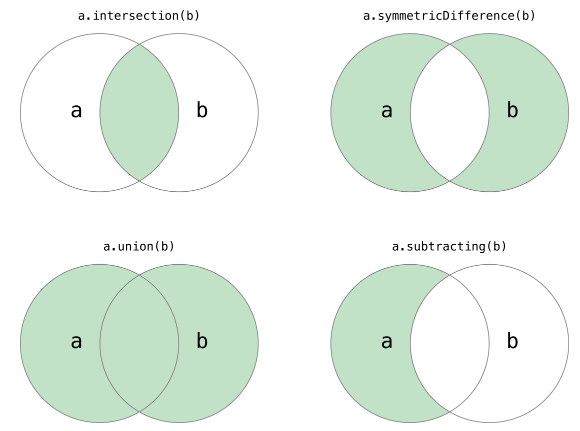# Python开发系列课程(8) - 字符串和常用数据结构

## 字符串和常用数据结构

### 使用字符串

$s={a}_{1}{a}_{2}\dots {a}_{n}\left(0\le n\le \mathrm{\infty }\right)$

def main():
str1 = 'hello, world!'
# 通过len函数计算字符串的长度
print(len(str1))  # 13
# 获得字符串首字母大写的拷贝
print(str1.capitalize())  # Hello, world!
# 获得字符串变大写后的拷贝
print(str1.upper())  # HELLO, WORLD!
# 从字符串中查找子串所在位置
print(str1.find('or'))  # 8
print(str1.find('shit'))  # -1
# 与find类似但找不到子串时会引发异常
# print(str1.index('or'))
# print(str1.index('shit'))
# 检查字符串是否以指定的字符串开头
print(str1.startswith('He'))  # False
print(str1.startswith('hel'))  # True
# 检查字符串是否以指定的字符串结尾
print(str1.endswith('!'))  # True
# 将字符串以指定的宽度居中并在两侧填充指定的字符
print(str1.center(50, '*'))
# 将字符串以指定的宽度靠右放置左侧填充指定的字符
print(str1.rjust(50, ' '))
str2 = 'abc123456'
# 从字符串中取出指定位置的字符(下标运算)
print(str2)  # c
# 字符串切片(从指定的开始索引到指定的结束索引)
print(str2[2:5])  # c12
print(str2[2:])  # c123456
print(str2[2::2])  # c246
print(str2[::2])  # ac246
print(str2[::-1])  # 654321cba
print(str2[-3:-1])  # 45
# 检查字符串是否由数字构成
print(str2.isdigit())  # False
# 检查字符串是否以字母构成
print(str2.isalpha())  # False
# 检查字符串是否以数字和字母构成
print(str2.isalnum())  # True
str3 = '  jackfrued@126.com '
print(str3)
# 获得字符串修剪左右两侧空格的拷贝
print(str3.strip())

if __name__ == '__main__':
main()


### 使用列表

def main():
list1 = [1, 3, 5, 7, 100]
print(list1)
list2 = ['hello'] * 5
print(list2)
# 计算列表长度(元素个数)
print(len(list1))
# 下标(索引)运算
print(list1)
print(list1)
# print(list1)  # IndexError: list index out of range
print(list1[-1])
print(list1[-3])
list1 = 300
print(list1)
# 添加元素
list1.append(200)
list1.insert(1, 400)
list1 += [1000, 2000]
print(list1)
print(len(list1))
# 删除元素
list1.remove(3)
if 1234 in list1:
list1.remove(1234)
del list1
print(list1)
# 清空列表元素
list1.clear()
print(list1)

if __name__ == '__main__':
main()


def main():
fruits = ['grape', 'apple', 'strawberry', 'waxberry']
fruits += ['pitaya', 'pear', 'mango']
# 循环遍历列表元素
for fruit in fruits:
print(fruit.title(), end=' ')
print()
# 列表切片
fruits2 = fruits[1:4]
print(fruits2)
# fruit3 = fruits  # 没有复制列表只创建了新的引用
# 可以通过完整切片操作来复制列表
fruits3 = fruits[:]
print(fruits3)
fruits4 = fruits[-3:-1]
print(fruits4)
# 可以通过反向切片操作来获得倒转后的列表的拷贝
fruits5 = fruits[::-1]
print(fruits5)

if __name__ == '__main__':
main()


def main():
list1 = ['orange', 'apple', 'zoo', 'internationalization', 'blueberry']
list2 = sorted(list1)
# sorted函数返回列表排序后的拷贝不会修改传入的列表
# 函数的设计就应该像sorted函数一样尽可能不产生副作用
list3 = sorted(list1, reverse=True)
# 通过key关键字参数指定根据字符串长度进行排序而不是默认的字母表顺序
list4 = sorted(list1, key=len)
print(list1)
print(list2)
print(list3)
print(list4)
# 给列表对象发出排序消息直接在列表对象上进行排序
list1.sort(reverse=True)
print(list1)

if __name__ == '__main__':
main()


import sys

def main():
f = [x for x in range(1, 10)]
print(f)
f = [x + y for x in 'ABCDE' for y in '1234567']
print(f)
# 用列表的生成表达式语法创建列表容器
# 用这种语法创建列表之后元素已经准备就绪所以需要耗费较多的内存空间
f = [x ** 2 for x in range(1, 1000)]
print(sys.getsizeof(f))  # 查看对象占用内存的字节数
print(f)
# 请注意下面的代码创建的不是一个列表而是一个生成器对象
# 通过生成器可以获取到数据但它不占用额外的空间存储数据
# 每次需要数据的时候就通过内部的运算得到数据(需要花费额外的时间)
f = (x ** 2 for x in range(1, 1000))
print(sys.getsizeof(f))  # 相比生成式生成器不占用存储数据的空间
print(f)
for val in f:
print(val)

if __name__ == '__main__':
main()


${F}_{0}=0$

${F}_{1}=1$

${F}_{n}={F}_{n-1}+{F}_{n-2}\left(n\ge 2\right)$def fib(n):
a, b = 0, 1
for _ in range(n):
a, b = b, a + b
yield a

def main():
for val in fib(20):
print(val)

if __name__ == '__main__':
main()


### 使用元组

Python 的元组与列表类似，不同之处在于元组的元素不能修改，在前面的代码中我们已经不止一次使用过元组了。顾名思义，我们把多个元素组合到一起就形成了一个元组，所以它和列表一样可以保存多条数据。下面的代码演示了如何定义和使用元组。

def main():
# 定义元组
t = ('骆昊', 38, True, '四川成都')
print(t)
# 获取元组中的元素
print(t)
print(t)
# 遍历元组中的值
for member in t:
print(member)
# 重新给元组赋值
# t = '王大锤'  # TypeError
# 变量t重新引用了新的元组原来的元组将被垃圾回收
t = ('王大锤', 20, True, '云南昆明')
print(t)
# 将元组转换成列表
person = list(t)
print(person)
# 列表是可以修改它的元素的
person = '李小龙'
person = 25
print(person)
# 将列表转换成元组
fruits_list = ['apple', 'banana', 'orange']
fruits_tuple = tuple(fruits_list)
print(fruits_tuple)

if __name__ == '__main__':
main()

1. 元组中的元素是无法修改的，事实上我们在项目中尤其是多线程环境（后面会讲到）中可能更喜欢使用的是那些不变对象（一方面因为对象状态不能修改，所以可以避免由此引起的不必要的程序错误，简单的说就是一个不变的对象要比可变的对象更加容易维护；另一方面因为没有任何一个线程能够修改不变对象的内部状态，一个不变对象自动就是线程安全的，这样就可以省掉处理同步化的开销。一个不变对象可以方便的被共享访问）。所以结论就是：如果不需要对元素进行添加、删除、修改的时候，可以考虑使用元组，当然如果一个方法要返回多个值，使用元组也是不错的选择。
2. 元组在创建时间和占用的空间上面都优于列表。我们可以使用sys模块的getsizeof函数来检查存储同样的元素的元组和列表各自占用了多少内存空间，这个很容易做到。我们也可以在ipython中使用魔法指令%timeit来分析创建同样内容的元组和列表所花费的时间，下图是我的macOS系统上测试的结果。### 使用集合

Python中的集合跟数学上的集合是一致的，不允许有重复元素，而且可以进行交集、并集、差集等运算。def main():
set1 = {1, 2, 3, 3, 3, 2}
print(set1)
print('Length =', len(set1))
set2 = set(range(1, 10))
print(set2)
set2.update([11, 12])
print(set1)
print(set2)
# remove的元素如果不存在会引发KeyError
if 4 in set2:
set2.remove(4)
print(set2)
# 遍历集合容器
for elem in set2:
print(elem ** 2, end=' ')
print()
# 将元组转换成集合
set3 = set((1, 2, 3, 3, 2, 1))
print(set3.pop())
print(set3)
# 集合的交集、并集、差集、对称差运算
print(set1 & set2)
# print(set1.intersection(set2))
print(set1 | set2)
# print(set1.union(set2))
print(set1 - set2)
# print(set1.difference(set2))
print(set1 ^ set2)
# print(set1.symmetric_difference(set2))
# 判断子集和超集
print(set2 <= set1)
# print(set2.issubset(set1))
print(set3 <= set1)
# print(set3.issubset(set1))
print(set1 >= set2)
# print(set1.issuperset(set2))
print(set1 >= set3)
# print(set1.issuperset(set3))

if __name__ == '__main__':
main()


### 使用字典

def main():
scores = {'骆昊': 95, '白元芳': 78, '狄仁杰': 82}
# 通过键可以获取字典中对应的值
print(scores['骆昊'])
print(scores['狄仁杰'])
# 对字典进行遍历(遍历的其实是键再通过键取对应的值)
for elem in scores:
print('%s\t--->\t%d' % (elem, scores[elem]))
# 更新字典中的元素
scores['白元芳'] = 65
scores['诸葛王朗'] = 71
scores.update(冷面=67, 方启鹤=85)
print(scores)
if '武则天' in scores:
print(scores['武则天'])
print(scores.get('武则天'))
# get方法也是通过键获取对应的值但是可以设置默认值
print(scores.get('武则天', 60))
# 删除字典中的元素
print(scores.popitem())
print(scores.popitem())
print(scores.pop('骆昊', 100))
# 清空字典
scores.clear()
print(scores)

if __name__ == '__main__':
main()


### 练习

#### 练习1：在屏幕上显示跑马灯文字

import os
import time

def main():
content = '北京欢迎你为你开天辟地…………'
while True:
# 清理屏幕上的输出
os.system('cls')  # os.system('clear')
print(content)
# 休眠200毫秒
time.sleep(0.2)
content = content[1:] + content

if __name__ == '__main__':
main()


#### 练习2：设计一个函数产生指定长度的验证码，验证码由大小写字母和数字构成。

import random

def generate_code(code_len=4):
"""
生成指定长度的验证码

:param code_len: 验证码的长度(默认4个字符)

:return: 由大小写英文字母和数字构成的随机验证码
"""
all_chars = '0123456789abcdefghijklmnopqrstuvwxyzABCDEFGHIJKLMNOPQRSTUVWXYZ'
last_pos = len(all_chars) - 1
code = ''
for _ in range(code_len):
index = random.randint(0, last_pos)
code += all_chars[index]
return code

#### 练习3：设计一个函数返回给定文件名的后缀名。

def get_suffix(filename, has_dot=False):
"""
获取文件名的后缀名

:param filename: 文件名
:param has_dot: 返回的后缀名是否需要带点

:return: 文件的后缀名
"""
pos = filename.rfind('.')
if 0 < pos < len(filename) - 1:
index = pos if has_dot else pos + 1
return filename[index:]
else:
return ''


#### 练习4：设计一个函数返回传入的列表中最大和第二大的元素的值。

def max2(x):
m1, m2 = (x, x) if x > x else (x, x)
for index in range(2, len(x)):
if x[index] > m1:
m2 = m1
m1 = x[index]
elif x[index] > m2:
m2 = x[index]
return m1, m2

#### 练习5：计算指定的年月日是这一年的第几天

def is_leap_year(year):
"""
判断指定的年份是不是闰年

:param year: 年份

:return: 闰年返回True平年返回False
"""
return year % 4 == 0 and year % 100 != 0 or year % 400 == 0

def which_day(year, month, date):
"""
计算传入的日期是这一年的第几天

:param year: 年
:param month: 月
:param date: 日

:return: 第几天
"""
days_of_month = [
[31, 28, 31, 30, 31, 30, 31, 31, 30, 31, 30, 31],
[31, 29, 31, 30, 31, 30, 31, 31, 30, 31, 30, 31]
][is_leap_year(year)]
total = 0
for index in range(month - 1):
total += days_of_month[index]

def main():
print(which_day(1980, 11, 28))
print(which_day(1981, 12, 31))
print(which_day(2018, 1, 1))
print(which_day(2016, 3, 1))

if __name__ == '__main__':
main()


#### 练习6：打印杨辉三角。

def main():
num = int(input('Number of rows: '))
yh = [[]] * num
for row in range(len(yh)):
yh[row] = [None] * (row + 1)
for col in range(len(yh[row])):
if col == 0 or col == row:
yh[row][col] = 1
else:
yh[row][col] = yh[row - 1][col] + yh[row - 1][col - 1]
print(yh[row][col], end='\t')
print()

if __name__ == '__main__':
main()


### 综合案例

#### 案例1：双色球选号

from random import randrange, randint, sample

def display(balls):
"""
输出列表中的双色球号码
"""
for index, ball in enumerate(balls):
if index == len(balls) - 1:
print('|', end=' ')
print('%02d' % ball, end=' ')
print()

def random_select():
"""
随机选择一组号码
"""
red_balls = [x for x in range(1, 34)]
selected_balls = []
for _ in range(6):
index = randrange(len(red_balls))
selected_balls.append(red_balls[index])
del red_balls[index]
# 上面的for循环也可以写成下面这行代码
# sample函数是random模块下的函数
# selected_balls = sample(red_balls, 6)
selected_balls.sort()
selected_balls.append(randint(1, 16))
return selected_balls

def main():
n = int(input('机选几注: '))
for _ in range(n):
display(random_select())

if __name__ == '__main__':
main()


#### 案例2：约瑟夫环问题

"""

《幸运的基督徒》

"""

def main():
persons = [True] * 30
counter, index, number = 0, 0, 0
while counter < 15:
if persons[index]:
number += 1
if number == 9:
persons[index] = False
counter += 1
number = 0
index += 1
index %= 30
for person in persons:
print('基' if person else '非', end='')

if __name__ == '__main__':
main()


#### 案例3：井字棋游戏

import os

def print_board(board):
print(board['TL'] + '|' + board['TM'] + '|' + board['TR'])
print('-+-+-')
print(board['ML'] + '|' + board['MM'] + '|' + board['MR'])
print('-+-+-')
print(board['BL'] + '|' + board['BM'] + '|' + board['BR'])

def main():
init_board = {
'TL': ' ', 'TM': ' ', 'TR': ' ',
'ML': ' ', 'MM': ' ', 'MR': ' ',
'BL': ' ', 'BM': ' ', 'BR': ' '
}
begin = True
while begin:
curr_board = init_board.copy()
begin = False
turn = 'x'
counter = 0
os.system('clear')
print_board(curr_board)
while counter < 9:
move = input('轮到%s走棋, 请输入位置: ' % turn)
if curr_board[move] == ' ':
counter += 1
curr_board[move] = turn
if turn == 'x':
turn = 'o'
else:
turn = 'x'
os.system('clear')
print_board(curr_board)
choice = input('再玩一局?(yes|no)')
begin = choice == 'yes'

if __name__ == '__main__':
main()©️2019 CSDN 皮肤主题: 编程工作室 设计师: CSDN官方博客